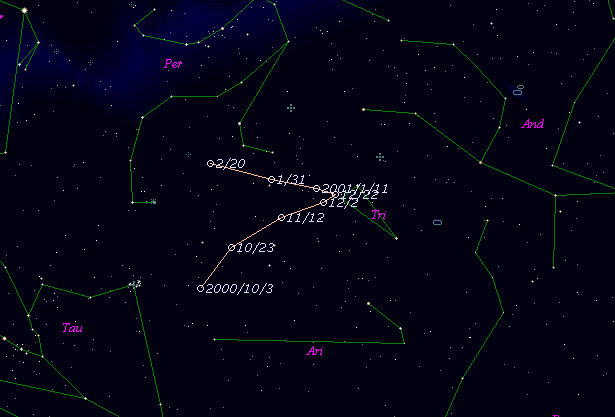# \$B%F%#%R!

196P/Tichy (2000)###\$B%W%m%U%#!<%k(B

 \$BId9f(B 196P/2000 U6 \$BH/8+F|(B 2000\$BG/(B10\$B7n(B23\$BF|(B \$BH/8+8wEY(B 17.8\$BEy(B \$BH/8+ Milos Tichy (Klet)

###\$B50F;MWAG(B

```                    Epoch = 2000 Oct. 23.0 TT
T = 2000 Oct.  4.6044 TT         Peri. =  11.8524
e = 0.431372                     Node  =  24.4340  2000.0
q = 2.154867 AU                  Incl. =  19.3690
a =  3.789587 AU    n = 0.1336030    P =   7.38 years
```

###\$B@1?^(B###\$B8wEYJQ2=(B

```        m1 = 12.5 + 5 log\$B&\$(B + 15.0 log r
```##### \$B50F;MWAG\$O!"(BIAUC 8917\$B\$K7G:\\$5\$l\$?\$b\$N\$G\$9!#(B \$B@1?^\$O(B StellaNavigator Ver.2.0 for Windows (\$B%"%9%H%m%"!<%D(B \$BJTCx(B / \$B%"%9%-!<=PHG6I4)(B) \$B\$G:n@.\$7\$?\$b\$N\$G\$9!#(B \$B8wEY%0%i%U\$O(BComet for Windows\$B\$G:n@.\$7\$?\$b\$N\$G\$9!#(B